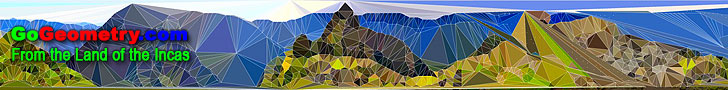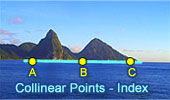# Recent additions (Page 17), Online LearningGo Geometry from the Land of the Incas - Recent Additions (Page 17)Geometry Problem 598 Trapezoid, Perpendicular, Equal Angles, Metric Relation. Geometry Problem 597 Quadrilateral, Right Triangle, Isosceles, Angle, Congruence. Geometry Problem 596 Quadrilateral, Right Triangle, Isosceles, Midpoint. Geometry Problem 595 Triangle, Equal Angles, Isogonal Conjugate, Similarity. Geometry Problem 594. Triangle, Incenter, Incircle, Inradius, Tangency Point, Midpoint, Altitude, Angle, Half the Difference. Geometry Problem 593. Triangle, Circumcircle, Collinear Points. Geometry Problems 581 - 590. Quadrilateral, Diagonals, Concurrency, Incenter, Metric Relations, Circumcenters, Parallelogram, Octagon, Areas, Tangency Point, Midpoint, Cyclic Quadrilateral, Volume of two Triangular Pyramids, Equal Trihedral Angle, Product of three Edges. Geometry Problem 592. Triangle, Incenter, Incircle, Tangency Point, Midpoints, Concurrent Lines, Congruence. Geometry Problem 591. Triangle, Incenter, Incircle, Inradius, Tangency Point, Midpoint, Altitude. Geometry Problem 590. Triangle, Incenter, Incircle, Tangency Point, Midpoint, Areas. Geometry Problem 589. Triangle, Incenter, Incircle, Tangency Points, Midpoint, Perpendicular, Distance. Geometry Problem 588. Triangle, Incenter, Incircle, Tangency Points, Midpoints, Distance, Sum. Geometry Problem 587. Triangle, Incenter, Incircle, Tangency Point, Midpoint, Distance, Half the Difference. Geometry Quote: John Jewel (1522-1571). English bishop of Salisbury. Geometry Problem 586. Cyclic Quadrilateral, Diagonals, Any Point, Circumcenters, Circumcircles, Concurrency. Go to Page: Previous | 1 | 2 | 3 | 4 | 5 | 6 | 7 | 8 | 9 | 10 | 11 | 12 | 13 | 14 | 15 | 16 | 17 | 18 | 19 | 20 | 21 | 22 | 23 | 24 | 25 | 26 | 27 | 28 | 29 | 30 | 31 | 32 | 33 | 34 | 35 | 36 | 37 | 38 | 39 | 40 | 41 | 42 | 43 | 44 | 45 | 46 | 47 | 48 | 49 | 50 | Next

 Home | GoGeometry Education | Geometry | Search | Email